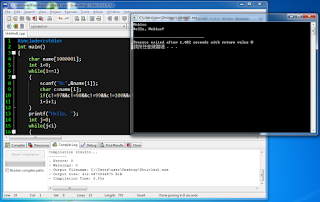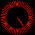## 2016年5月10日 星期二

### 給新手的C++教學 (上冊) - 8. 字元 & 字串 (Charactor & String)

1. 土法煉鋼法

#include<cstdio>
int main()
{
char name;
int i=0;
while(1==1)
{
scanf("%c",&name[i]);
char c=name[i];
if(c!='a'&&c!='b'&&c!='c'&&c!='d'&&c!='e'&&c!='f'&&c!='g'&&c!='h'&&c!='i'&&c!='j'&&c!='k'&&c!='l'&&c!='m'&&c!='n'&&c!='o'&&c!='p'&&c!='q'&&c!='r'&&c!='s'&&c!='t'&&c!='u'&&c!='v'&&c!='w'&&c!='x'&&c!='y'&&c!='z'&&c!='A'&&c!='B'&&c!='C'&&c!='D'&&c!='E'&&c!='F'&&c!='G'&&c!='H'&&c!='I'&&c!='J'&&c!='K'&&c!='L'&&c!='M'&&c!='N'&&c!='O'&&c!='P'&&c!='Q'&&c!='R'&&c!='S'&&c!='T'&&c!='U'&&c!='V'&&c!='W'&&c!='X'&&c!='Y'&&c!='Z')break;
i=i+1;
}
printf("Hello, ");
int j=0;
while(j<i)
{
printf("%c",name[j]);
j=j+1;
}
printf("!\n");
return 0;
}

52個字母一一判斷實在是太累了，而且容易打錯

#include<cstdio>
int main()
{
printf("%d %d\n",'M','o');
}程式輸出「77 111」了！

#include<cstdio>
int main()
{
char name;
int i=0;
while(1==1)
{
scanf("%c",&name[i]);
char c=name[i];
if(c!=97&&c!=98&&c!=99&&c!=100&&c!=101&&c!=102&&c!=103&&c!=104&&c!=105&&c!=106&&c!=107&&c!=108&&c!=109&&c!=110&&c!=111&&c!=112&&c!=113&&c!=114&&c!=115&&c!=116&&c!=117&&c!=118&&c!=119&&c!=120&&c!=121&&c!=122&&c!=65&&c!=66&&c!=67&&c!=68&&c!=69&&c!=70&&c!=71&&c!=72&&c!=73&&c!=74&&c!=75&&c!=76&&c!=77&&c!=78&&c!=79&&c!=80&&c!=81&&c!=82&&c!=83&&c!=84&&c!=85&&c!=86&&c!=87&&c!=88&&c!=89&&c!=90)break;
i=i+1;
}
printf("Hello, ");
int j=0;
while(j<i)
{
printf("%c",name[j]);
j=j+1;
}
printf("!\n");
return 0;
}輸入「Mobius」，程式依然是輸出「Hello, Mobius!」 (順帶一提，「Mobius」是我們「105級高雄中學科學班成果發表會」的名稱，歡迎大家前去看看~~~)

2. 邏輯判斷法

「'a'~'z'」對應到數字「97~122」
「'A'~'Z'」對應到數字「65~90」

#include<cstdio>
int main()
{
char name;
int i=0;
while(1==1)
{
scanf("%c",&name[i]);
char c=name[i];
if((97<=c&&c<=122)||(65<=c&&c<=90));
else break;
i=i+1;
}
printf("Hello, ");
int j=0;
while(j<i)
{
printf("%c",name[j]);
j=j+1;
}
printf("!\n");
return 0;
}

「判斷c是字母」比「判斷c不是字母」還要來得容易

#include<cstdio>
int main()
{
char name;
int i=0;
while(1==1)
{
scanf("%c",&name[i]);
char c=name[i];
if(!((97<=c&&c<=122)||(65<=c&&c<=90))) break;
i=i+1;
}
printf("Hello, ");
int j=0;
while(j<i)
{
printf("%c",name[j]);
j=j+1;
}
printf("!\n");
return 0;
}

#include<cstdio>
int main()
{
char name;
int i=0;
while(1==1)
{
scanf("%c",&name[i]);
char c=name[i];
if(!(('a'<=c&&c<='z')||('A'<=c&&c<='Z'))) break;
i=i+1;
}
printf("Hello, ");
int j=0;
while(j<i)
{
printf("%c",name[j]);
j=j+1;
}
printf("!\n");
return 0;
}趕快驗證一下，程式還是在做同樣一件事情，對吧？

3. 投機取巧法

「隱藏字元」泛指「無法直接顯示出來」的字元，例如：空白「' '」、Tab「'\t'」、換行「'\n'」

#include<cstdio>
int main()
{
char name;
scanf("%s",name);
printf("Hello, ");
printf("%s",name);
printf("!\n");
return 0;
}

#include<cstdio>
int main()
{
char name;
scanf("%s",name);
printf("Hello, %s!\n",name);
return 0;
}趕快驗證一下，程式還是在做同樣一件事情，對吧？

#### 8 則留言:

1.好像有bug啊啊

1.您好，請問bug是什麼呢？
小莫很樂意修正！
感謝~

2.那一整串的運算元都超出螢幕拉

3.當然如果那是故意的就沒差就是了

4.這個嘛...
剛剛針對這個問題上網研究了一下，還是找不到有用的解決方法
抱歉哦...QQ

2.嗨 百五的雄中學長,其實上面顯型字元,有包括數字
,所以應該還是有差啦 ,XD ~

1.恩對呀XD
那您有建議要怎麼修改嗎？

3.想問一下 我在程式上開設一個 char name 長度為10的char
接著用cin附值給他(我故意將附值內容多於10個字)
接著用cout來print name時可以完全輸出我剛剛打的名字
但用sizeof(name)時name的byte還是為10，這是什麼原因呢?